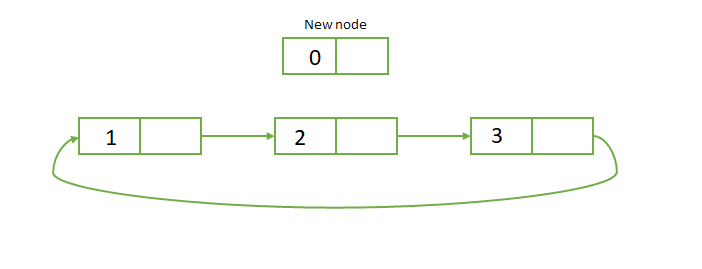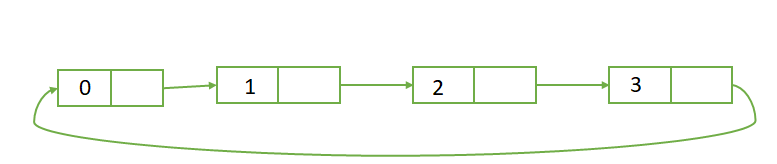GeeksforGeeks App
Open AppBrowser
Continue

# Java Program to Insert a New Node at the Beginning of the Circular Linked List

Circular linked list: A circular linked list is a sequence of elements in which every element points to its next element in the sequence and the last element has a link to the first element. That means a circular linked list is similar to the single linked list except that the last node points to the first node in the list.

Inserting a new node at the beginning of the circular linked list:

If the linked list is empty then both head and tail will point to the newly added node. If the list is not empty, then we will point the last node to newly added node and point the newly added node to head node and finally, we make the newly added node as the head node.Algorithm :

1. Create a Node class which represents a node in the list. It has two variables data and next pointer(which points to the next node).
2. Create another class for creating the circular linked list and it has two nodes namely head and tail.
3. When adding a new node to list then, we will first check whether the head is null. If the list is empty or head is null then we will insert the node as the head, and tail also points to the newly added node.
4. If the list is not empty, then the newly added node will point to head, and the tail will point to a newly added node and New node will be made as to the head node.

Code Snippet :

## Java

 `// Java Program to Insert a nodes at the Beginning of the``// Circular Linked List` `public` `class` `AddAtBeginning {` `    ``// Represents the node of list.``    ``public` `class` `Node {``        ``char` `data;``        ``Node next;``        ``public` `Node(``char` `data) { ``this``.data = data; }``    ``}` `    ``// Declaring head and tail pointer as null.``    ``// head indicates the starting node and tail indicates``    ``// last node.``    ``public` `Node head = ``null``;``    ``public` `Node tail = ``null``;` `    ``// This function will add the new node at the Beginning``    ``// of the circular linked list.``    ``public` `void` `addNode(``char` `data)``    ``{``        ``// Create new node``        ``Node newNode = ``new` `Node(data);` `        ``// Checks if the list is empty.``        ``if` `(head == ``null``) {` `            ``// make newnode as both head and tail node.``            ``head = newNode;``            ``tail = newNode;` `            ``// point the tail to head node.``            ``tail.next = head;``        ``}``        ``else` `{` `            ``// point the newnode to head``            ``newNode.next = head;` `            ``// point the rail to new node.``            ``tail.next = newNode;` `            ``// make the newnode as head.``            ``head = newNode;``        ``}``    ``}` `    ``// printLinkedList prints all the nodes in the list``    ``public` `void` `printLinkedList()``    ``{``        ``Node presentNode = head;``        ``if` `(head == ``null``) {``            ``System.out.println(``"List is empty"``);``        ``}``        ``else` `{``            ``System.out.println(``"\n"``);` `            ``// here with out checking anything we will print``            ``// the first node, And print the rest of the``            ``// nodes till the pointer again reaches the head``            ``// node.``            ``do` `{``                ``System.out.print(``" "` `+ presentNode.data);` `                ``// incrementing the nodes using next``                ``// property.``                ``presentNode = presentNode.next;` `                ``// if present node is head, stop printing``                ``// the nodes.``            ``} ``while` `(presentNode != head);``        ``}``    ``}` `    ``public` `static` `void` `main(String[] args)``    ``{``        ``AddAtBeginning obj = ``new` `AddAtBeginning();` `        ``System.out.println(``            ``"Adding nodes at the beginning of the list: "``);``        ``obj.addNode(``'s'``);``        ``obj.printLinkedList();` `        ``// add k at the beginning``        ``obj.addNode(``'k'``);``        ``obj.printLinkedList();` `        ``// add e at the beginning``        ``obj.addNode(``'e'``);``        ``obj.printLinkedList();` `        ``// add e at the beginning``        ``obj.addNode(``'e'``);``        ``obj.printLinkedList();` `        ``// add G at the beginning``        ``obj.addNode(``'G'``);``        ``obj.printLinkedList();``    ``}``}`

Output

```Adding nodes at the beginning of the list:

s

k s

e k s

e e k s

G e e k s```

Time Complexity: O(1)

Auxiliary space: O(1) as it is using constant space

My Personal Notes arrow_drop_up